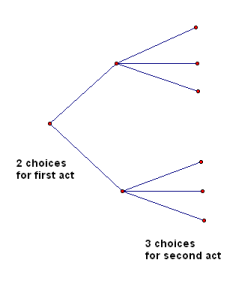﻿ More Probability Concepts
MORE PROBABILITY CONCEPTS

## Properties of Probabilities

Recall that the probability of an event $\,E\,$ is denoted by $\,P(E)\,$.

• For any event $\,E\,$, $\,0\le P(E)\le 1\,$.
That is, a probability is always a number between $\,0\,$ and $\,1\,$.
• If $\,S\,$ is the sample space, then $\,P(S)=1\,$.
That is, there's a $\,100\%\,$ chance that something will happen.
• For a finite sample space (i.e., an experiment with a finite number of outcomes):
a probability of $\,1\,$ means the event will definitely occur;
a probability of $\,0\,$ means the event will definitely not occur.

Notice that if the experiment is to randomly choose a real number from the interval $\,[0,1]\,$,
then the probability of choosing (say) the number $\,0.5\,$ is zero,
and yet this outcome could occur.
This is why a finite sample space is needed in the above statement.
• For any events $\,A\,$ and $\,B\,$: $$P(A\cup B)=P(A)+P(B)-P(A\cap B)$$ If you were just to add the two probabilities, then the intersection gets added twice; this extra probability must be subtracted.
• Recall that $\,\overline{E}\,$ denotes the complement of $\,E\,$.
For any event, either it happens, or it doesn't!
Therefore, $\,P(E)+P(\overline{E})=1\,$.
Equivalently, $\,P(\overline{E})=1 -P(E)\,$.
• Recall that $\,\emptyset \,$ denotes the empty set.
Events $\,A\,$ and $\,B\,$ are said to be mutually exclusive if $\,A\cap B = \emptyset\,$.
That is, two events are mutually exclusive when they have nothing in common (their intersection is empty).
Since $\,P(\emptyset) = 0\,$, for mutually exclusive events, the above formula is simpler: $$\,P(A\cup B)=P(A)+P(B)\,$$
 MULTIPLICATION COUNTING PRINCIPLE If there are $\,F\,$ choices for how to perform a first act, and for each of these $\,F\,$ ways, there are $\,S\,$ choices for how to perform a second act, then there are $\,F\cdot S\,$ ways to perform the acts in succession. (The idea extends to more than $\,2\,$ acts.) The idea is illustrated by the diagram at right. If there are $\,2\,$ piles, with $\,3\,$ in each pile, then the total is $\,2\cdot 3 = 6\,$.EXAMPLE (pizza choices)
Suppose that a pizza shop offers $\,3\,$ types of crust,
$\,2\,$ different types of cheese, and $\,7\,$ different toppings.

A single-topping pizza consists of a choice of crust,
a choice of cheese (if desired), and a choice of topping.

How many different single-topping pizzas are there?
Solution:
There are $\,3\,$ choices for the crust,
$\,3\,$ choices for cheese (none, first type, second type),
and $\,7\,$ choices for the single topping.

By the Multiplication Counting Principle, there are $\,3\cdot 3\cdot 7 = 63\,$ possible single-topping pizzas.
Master the ideas from this section